### Python 并发编程

1. CPU 密集型与 I/O 密集型

2. 多线程，多进程和多协程的对比

1. python 速度慢的两大原因

2. GIL 是什么？

3. 为什么会有GIL 这个东西？

4. 怎么样规避GIL 带来的限制？

1. python 创建线程的方法；

2. 改写spider程序，变成多线程爬取；

1. 多组件的Pipeline 技术架构

2. 生产者消费者的爬虫架构

3. 生产者消费者爬虫

1. 线程安全的概念

2. python 可以使用 Lock 类来解决线程安全问题

1. 线程池的原理

2. 使用线程池的好处

1. web 服务的架构以及特点

# 一. 为什么需要引入并发编程呢？

① 单线程串行：由CPU 和 IO 轮流执行；② 多线程并发（threading）③ 多CPU 并行（multiprocessing）④ 多机器并行；

python 对于并发编程的支持：

1. 多线程：threading，利用CPU 和 IO 可以同时执行的原理，让CPU 不会干巴巴等待IO 完成；
2. 多进程：multiprocessing，利用多核CPU的能力，真正并行执行任务；
3. 异步IO：asyncio，在单线程利用CPU 和 IO 同时执行的原理，实现函数的异步执行；
4. 使用 Lock 对资源进行加锁，防止冲突访问；python 也提供了Queue 实现不同线程/进程之间的数据通信 ，实现生产者–消费者模式
5. 使用线程池Pool/进程池Pool，简化线程/进程任务提交，等待结果、获取结果；
6. 使用subprocess 启动外部程序的进程，并进行输入输出的交互；

# 二. 怎么样选择多线程，多进程和多协程？

python 并发编程有三种模式：1. 多线程Thread；2. 多进程Process；3. 多协程Corroutine；思考一下三个问题：1. 什么是CPU密集型计算，IO 密集型计算；2. 多线程，多进程和多协程的区别；3. 怎么样根据任务选择对应技术？

## 1. CPU 密集型与 I/O 密集型

CPU 密集型（CPU-bound）：CPU 密集型也叫做计算密集型，是指I/O在很短时间内就可以完成，CPU 需要大量的计算和处理，特点是CPU 占用率相当高；例如：压缩解压缩、加密解密、正则表达式搜索；I/O密集型（I/O bound）：IO 密集型指的是系统运作大部分的状况是CPU 在等I/O（硬盘，内存）的读写操作，CPU 占用率较低，例如：文件处理程序，网络爬虫程序，读写数据库程序；

# 三. python 的全局解释器锁（GIL）

## 2. GIL 是什么？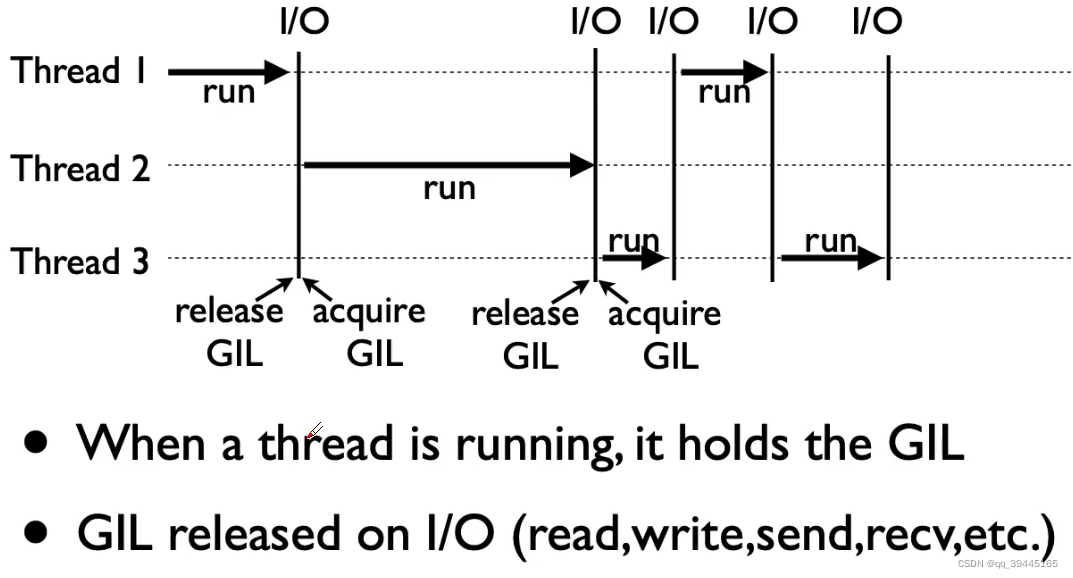# 四. 使用多线程加速python spider

## 2. 改写spider程序，变成多线程爬取；

blog_spider.py：

``import requests # urls存储爬取页面的url, 以f开头表示在字符串内支持大括号内的python表达式(列表表达式生成列表的每一个元素)urls = [f"https://www.cnblogs.com/#p{page}" for page in range(1, 51)]  # 爬取函数def craw(url: str):    # get()方法给url 对应的服务器发送一个get请求, 返回值为Response 类    r = requests.get(url)    print(url, len(r.text))``

``import threadingimport time import blog_spider  def single_thread():    print("single-thread begin")    for url in blog_spider.urls:        blog_spider.craw(url)    print("single-thread end")  def multi_thread():    print("multi-thread begin")    # threads 存储线程对象    threads = list()    for url in blog_spider.urls:        threads.append(            # target 传递的是调用的函数, 当前调用的函数需要开启线程来处理, args为函数的参数, 需要传递一个元祖类型            threading.Thread(target=blog_spider.craw, args=(url,))        )    for thread in threads:        # 使用start()启动线程        thread.start()    # 使用join()函数等待结束    for thread in threads:        thread.join()    print("multi-thread end")  if __name__ == "__main__":    # 计算使用单线程爬取的耗时    start = time.time()    single_thread()    end = time.time()    print("single-thread cost: ", end - start, " seconds")        # 计算使用多线程爬取的耗时    start = time.time()    multi_thread()    end = time.time()    print("multi-thread cost: ", end - start, " seconds")``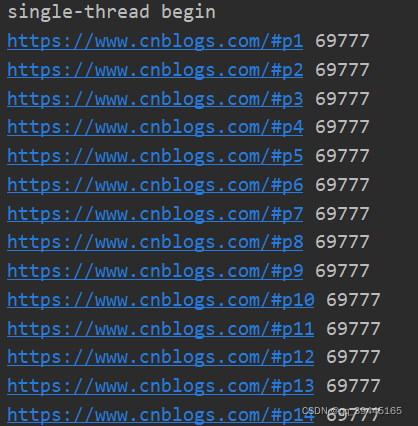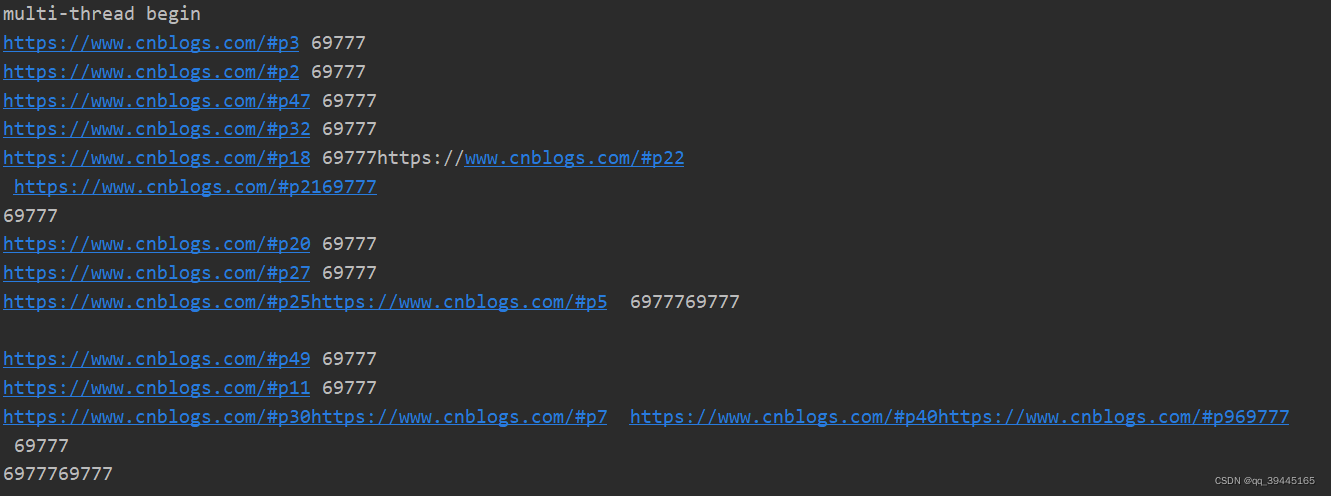# 五. 生产消费者spider

## 1. 多组件的Pipeline 技术架构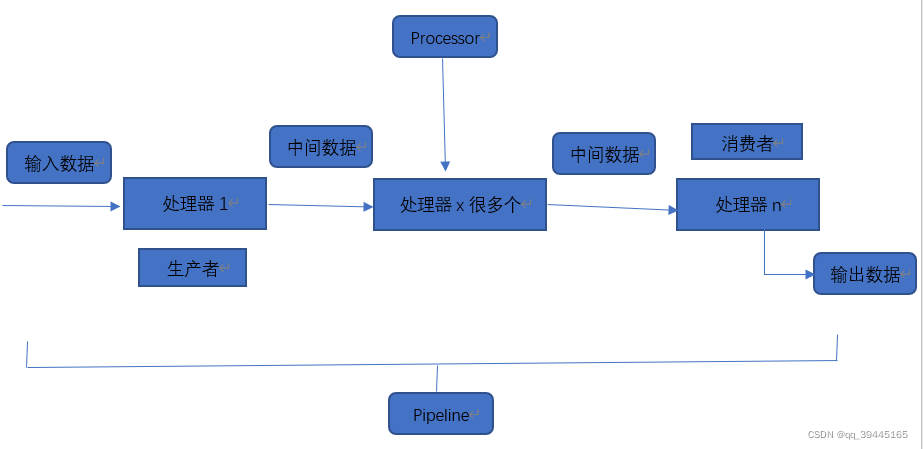## 2. 生产者消费者的爬虫架构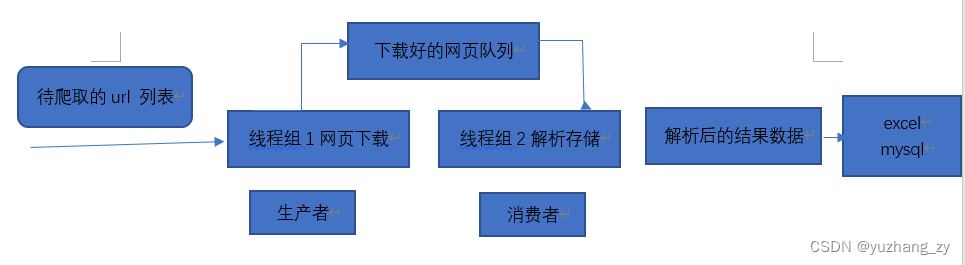3. 多线程数据通信的queue.Queue

queue.Queue 可以用于多线程之间的，线程安全的数据通信

``import queue q = queue.Queue()# 添加元素, 当队列满的时候会一直等待直到有人取出元素q.put(elem)# 当队列为空的时候使用get()会一直阻塞直到队列中有元素之后elem = q.get()``

## 3. 生产者消费者爬虫

``import requestsfrom bs4 import BeautifulSoup # urls存储爬取页面的url, 以f开头表示在字符串内支持大括号内的python表达式urls = [f"https://www.cnblogs.com/#p{page}" for page in range(1, 51)]  # 爬取函数def craw(url: str):    r = requests.get(url)    return r.text  def parse(html):    soup = BeautifulSoup(html, "html.parser")    # links = soup.find_all("a", "post-item-title")    # 当html 文档需要获取对应的css属性对应的标签的时候这个时候可以使用 css属性名字_= "属性值"的方式    links = soup.find_all("a", class_="post-item-title")    return [(link["href"], link.get_text()) for link in links]``

producer_consumer_spider.py：

``import queueimport blog_spiderimport timeimport randomimport threading # do_craw方法用来获取url_queue队列中url的值并爬取url地址对应的html内容并将其放入到html_queue队列中def do_craw(url_queue: queue.Queue, html_queue: queue.Queue):    while True:        url = url_queue.get()        html = blog_spider.craw(url)        html_queue.put(html)        print(threading.current_thread().name, f"craw {url}", "url_queue.size=", url_queue.qsize())        time.sleep(random.randint(1, 2)) # do_parse方法用来获取爬取的html_queue的html内容并将解析, 将解析的结果写入到文件中def do_parse(html_queue: queue.Queue, fout):    while True:        html = html_queue.get()        results = blog_spider.parse(html)        for res in results:            fout.write(str(res) + "\n")        print(threading.current_thread().name, f"result.size", len(results), "html_queue.size=", html_queue.qsize())        time.sleep(random.randint(1, 2))  if __name__ == '__main__':    url_queue = queue.Queue()    html_queue = queue.Queue()    for url in blog_spider.urls:        url_queue.put(url)    fout = open("data.txt", "w")    # 开启三个线程来处理这些爬取html的函数    for idx in range(3):        t = threading.Thread(target=do_craw, args=(url_queue, html_queue), name=f"craw{idx}")        t.start()    # 开启两个线程解析爬取的html页面的内容    for idx in range(2):        t = threading.Thread(target=do_parse, args=(html_queue, fout), name=f"parse{idx}")        t.start()``

# 六. python 线程安全问题以及解决方案

## 2. python 可以使用 Lock 类来解决线程安全问题

``# 用法1import threading lock = threading.Lock()lock.acquire()try:    ... finally:    lock.release() # 用法2import threadinglock = threading.Lock()with lock:    ...``

``import threadingimport time  class Account:    def __init__(self, balance):        self.balance = balance  def draw(account, amount):    if account.balance >= amount:        # 调用sleep()大概率会出现问题, 因为此时会发生线程的切换, 那么就一定会进入if判断的语句那么两个线程都会balance 操作就出现了问题        time.sleep(0.1)        print(threading.current_thread().name + "取钱成功")        account.balance -= amount        print(threading.current_thread().name + " 余额: ", account.balance)     else:        print(threading.current_thread().name, "取钱失败, 余额不足")  if __name__ == '__main__':    account = Account(1000)    # 使用线程a, b来执行draw 函数    ta = threading.Thread(name="a", target=draw, args=(account, 800))    tb = threading.Thread(name="b", target=draw, args=(account, 800))    # 使用start()会启动一个线程    ta.start()    tb.start()``
``# 有可能输出错误的结果, 也有可能输出正确的结果a取钱成功b取钱成功b 余额:  200a 余额:  -600 # 不够大部分的情况下输出的答案都是正确的, 这就是多个线程共同操作共享变量的时候发生的线程安全问题 a取钱成功a 余额:  200b 取钱失败, 余额不足``

``import threadingimport time # 获取锁对象lock = threading.Lock()  class Account:    def __init__(self, balance):        self.balance = balance  def draw(account, amount):    # 使用with关键字对发生线程安全的代码进行加锁, 当一个线程执行完成之后才会释放锁另外一个线程才可以获取到锁    with lock:        if account.balance >= amount:            # 调用sleep()大概率会出现问题            time.sleep(0.1)            print(threading.current_thread().name + "取钱成功")            account.balance -= amount            print(threading.current_thread().name + " 余额: ", account.balance)         else:            print(threading.current_thread().name, "取钱失败, 余额不足")  if __name__ == '__main__':    account = Account(1000)    ta = threading.Thread(name="a", target=draw, args=(account, 800))    tb = threading.Thread(name="b", target=draw, args=(account, 800))    ta.start()    tb.start()``

## 1. 线程池的原理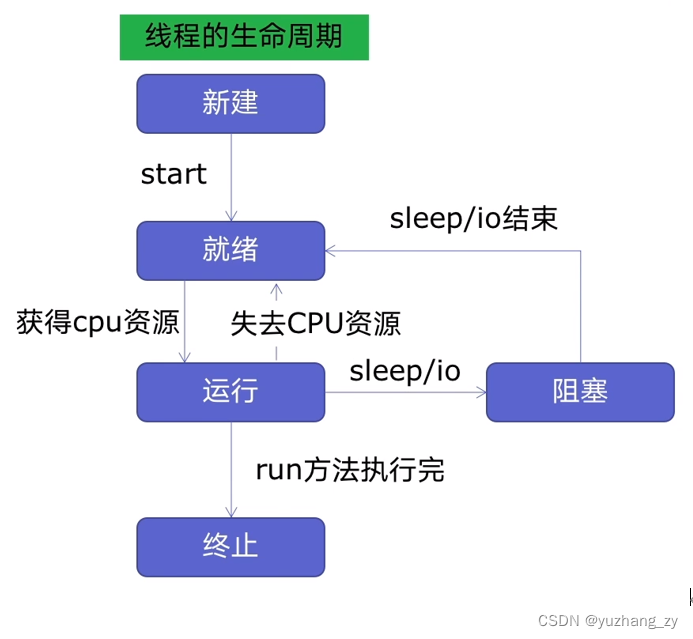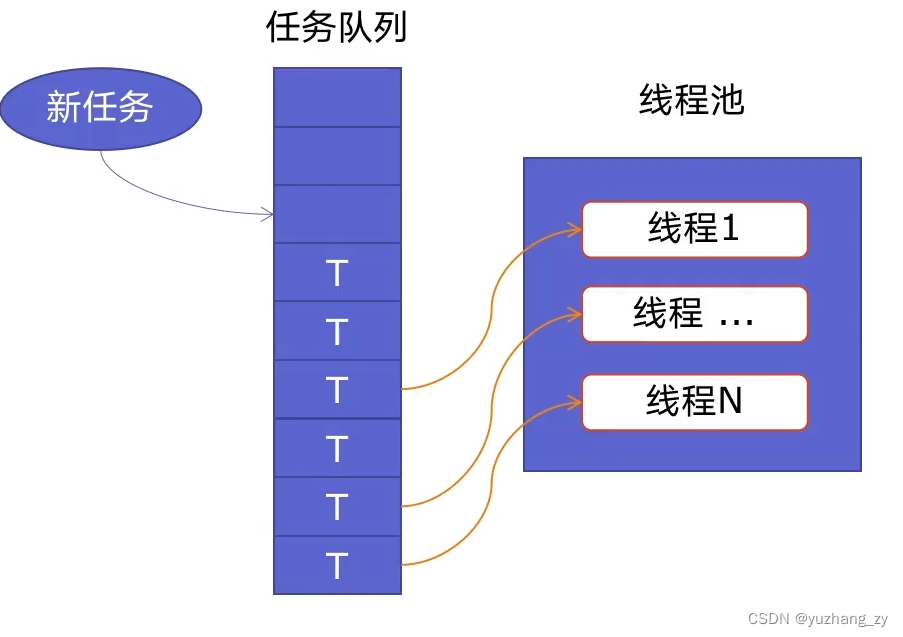## 2. 使用线程池的好处

① 提升了性能：因为减去了大量新建，终止线程的开销，重用了线程资源；② 适用场景：处理处理突发性大量请求或者需要大量线程完成任务，但是实际上任务处理时间较短；③ 防御功能：能够有效避免系统因为创建线程过多，而导致的系统负荷过大相应变慢等问题；

``import concurrent.futuresimport blog_spider with concurrent.futures.ThreadPoolExecutor() as pool:    htmls = pool.map(blog_spider.craw, blog_spider.urls)    htmls = list(zip(blog_spider.urls, htmls))    for url, html in htmls:        print(url, len(html))print("craw over") with concurrent.futures.ThreadPoolExecutor() as pool:    futures = dict()    # 使用submit的方式    for url, html in htmls:        future = pool.submit(blog_spider.parse, html)        # future与url建立一个关系        futures[future] = url    for future, url in futures.items():        print(url, future.result())    # as_completed 哪一个任务先执行完就返回哪一个结果    # for future in concurrent.futures.as_completed(futures):    #     url = futures[future]    #     print(url, future.result())print("parse over")``

# 八. 在web 服务中使用线程池加速

## 1. web 服务的架构以及特点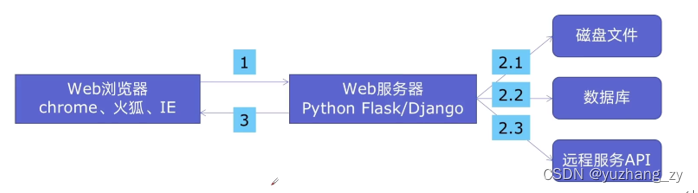web 后台服务的特点：

① web 服务对响应时间要求非常高，比如要求200ms 返回；② web 服务有大量的依赖IO操作的调用，比如磁盘文件，数据库，远程API（可以使用线程池的技术加速）；③ web 服务经常需要处理几万人，几百万人同时请求；

① 方便将磁盘文件，数据库，远程API的IO 调用并发执行；② 线程池的线程数目不会无限创建（导致系统挂掉）；具有防御功能；

``import jsonimport time import flask app = flask.Flask(__name__) # 使用time.sleep()方法模拟读取文件的操作, 其余两个方法也是类似的def read_file():    time.sleep(0.1)    return "file_read result"  def read_db():    time.sleep(0.2)    return "db_read result"  def read_api():    time.sleep(0.6)    return "api_read result" # 映射的url@app.route("/")def index():    result_file = read_file()    result_db = read_db()    result_api = read_api()    #返回json字符串    return json.dumps({        "result_file": result_file,        "result_db": result_db,        "result_api": result_api    })  if __name__ == '__main__':    # 启动flask 服务    app.run()``

``import jsonimport timefrom concurrent.futures import ThreadPoolExecutorimport flask# 创建一个全局的poolpool = ThreadPoolExecutor() app = flask.Flask(__name__)  def read_file():    time.sleep(0.1)    return "file_read result"  def read_db():    time.sleep(0.2)    return "db_read result"  def read_api():    time.sleep(0.6)    return "api_read result"  @app.route("/")def index():    # 使用submit()函数返回的是Future对象    result_file = pool.submit(read_file)    result_db = pool.submit(read_db)    result_api = pool.submit(read_api)    return json.dumps({        "result_file": result_file.result(),        "result_db": result_db.result(),        "result_api": result_api.result()    })  if __name__ == '__main__':    # 启动flask 服务    app.run()``

# 九. 使用多进程 multiprocessing 加速程序的运行

1. 有了多线程threading，为什么还要用多进程multiprocessing，虽然对于IO 密集型来说多线程确实可以实现加速的效果，因为当遇到IO的时候cpu会切换到另外的线程，此时当前的线程可以执行当前的IO操作，这样就实现了多线程加速的效果，但是当遇到的是cpu 密集型来说，没有IO的操作，当一个线程执行一段时间之后那么切换到其他的线程，此时会发生线程切换的消耗，非但没有实现加速的效果，返回减慢了运行速度；而multiprocessing 模块就是为了python 为了解决GIL 缺陷而引入的一个模块，原理是在用多进程在多cpu 上并行执行；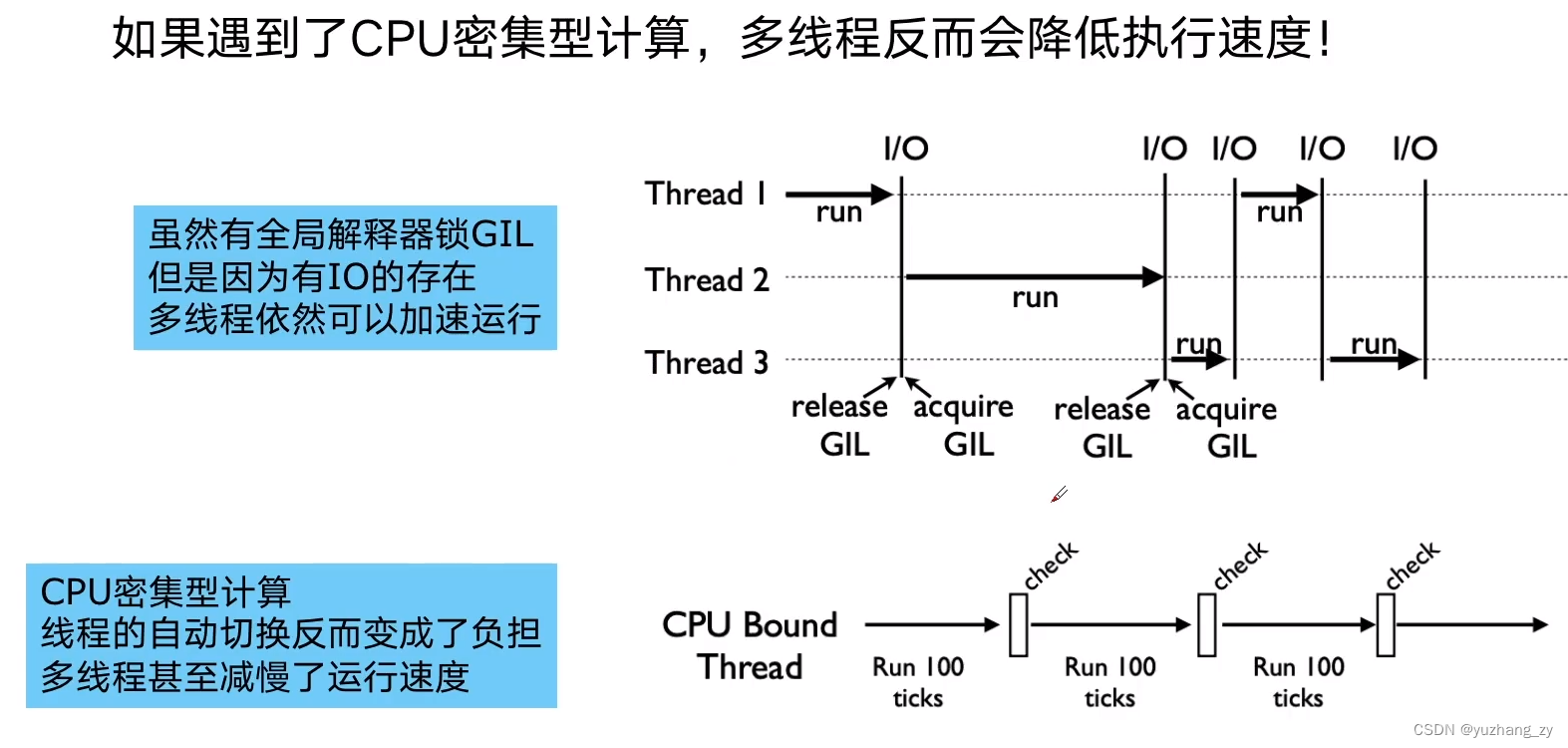2. 多进程multiprocessing 知识梳理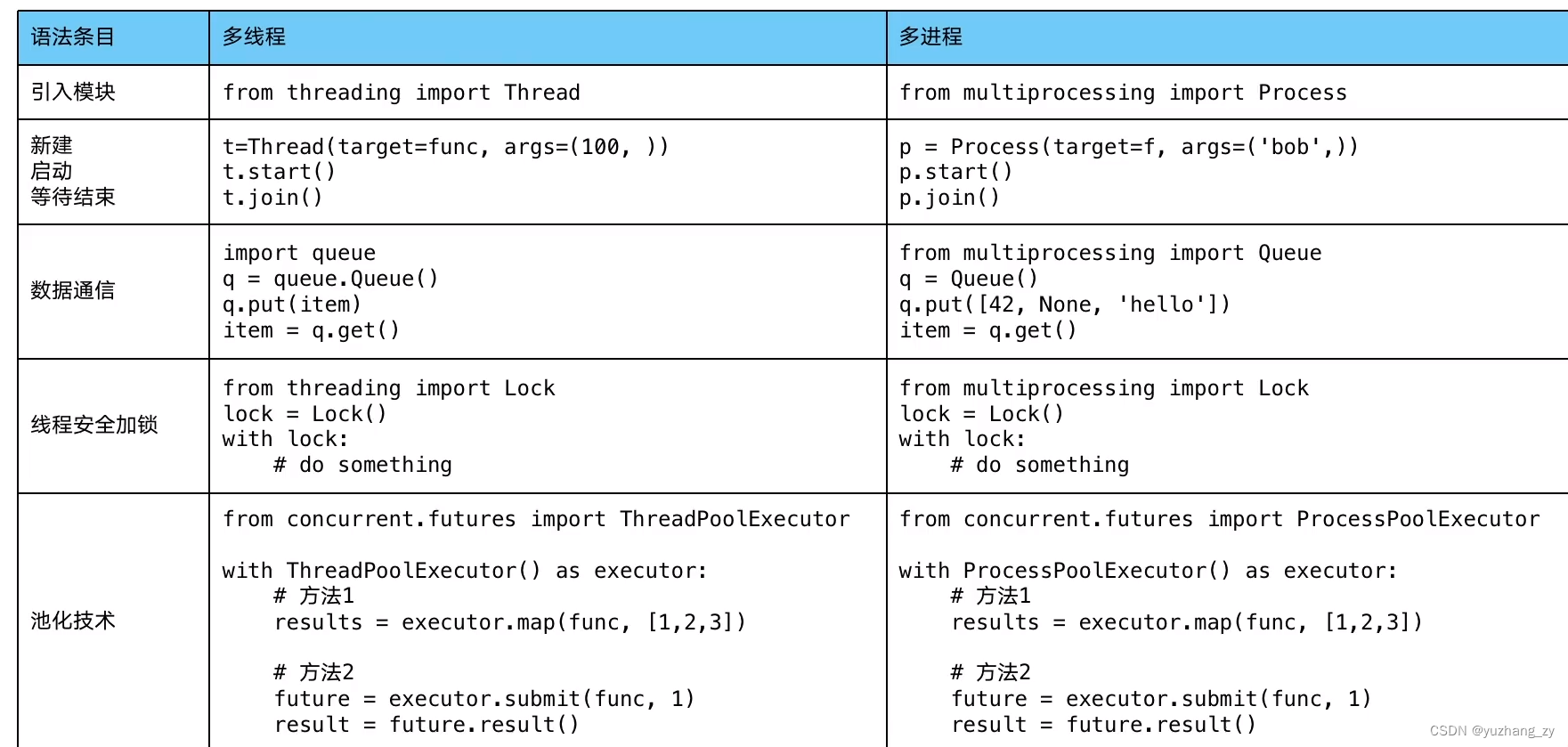3. 单线程，多线程，多进程对比 CPU密集型计算速度

cpu 密集型计算：100次判断大数字是否是素数的计算，由于GIL 的存在，多线程比单线程计算还慢，而多进程可以明显加快执行速度（实际运行结果可能有的时候有偏差但是几乎单线程与多线程的计算速度差不多，多进程是最快的）：

``from concurrent.futures import ThreadPoolExecutor, ProcessPoolExecutorimport time  class Solution:    primes =  * 100     # 判断n是否是素数    def is_prime(self, n: int):        i = 2        while i * i <= n:            if n % i == 0: return False            i += 1        return True     def single_thread(self):        for x in self.primes:            self.is_prime(x)     def multi_thread(self):        with ThreadPoolExecutor() as pool:            pool.map(self.is_prime, self.primes)     def multi_process(self):        with ProcessPoolExecutor() as pool:            pool.map(self.is_prime, self.primes)  if __name__ == '__main__':    start = time.time()    Solution().single_thread()    end = time.time()    print("single_thread cost", end - start, "s")     start = time.time()    Solution().multi_thread()    end = time.time()    print("multi_thread cost", end - start, "s")     start = time.time()    Solution().multi_process()    end = time.time()    print("multi_process cost", end - start, "s")``
``single_thread cost 185.69155049324036 smulti_thread cost 181.973712682724 smulti_process cost 87.62334203720093 s``

``import flaskfrom concurrent.futures import ProcessPoolExecutorimport json app = flask.Flask(__name__)  def is_prime(n: int):    i = 2    while i * i <= n:        if n % i == 0: return False        i += 1    return True  @app.route("/is_prime/<numbers>")def api_is_prime(numbers):    number_list = [int(x) for x in numbers.split(",")]    results = process_pool.map(is_prime, number_list)    return json.dumps(dict(zip(number_list, results)))  # 下面直接运行会报错if __name__ == '__main__':    process_pool = ProcessPoolExecutor()    app.run()``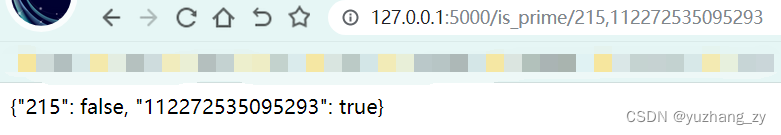# 十一. python 异步IO 实现并发spider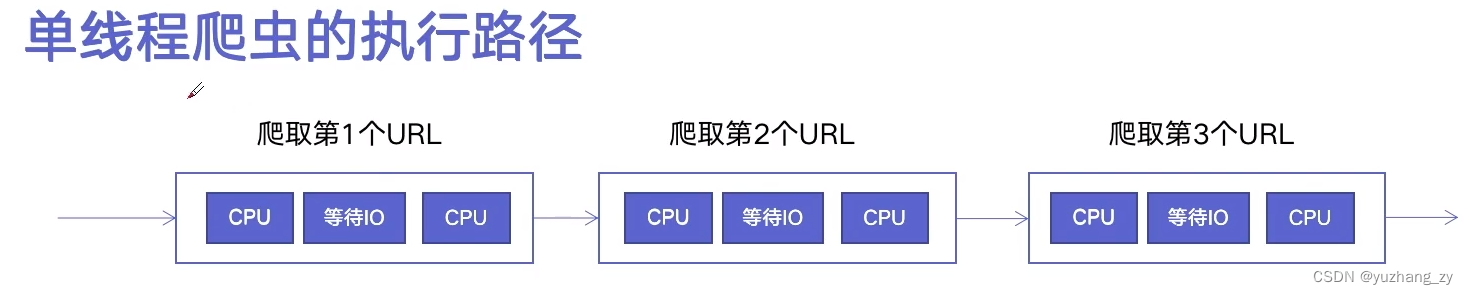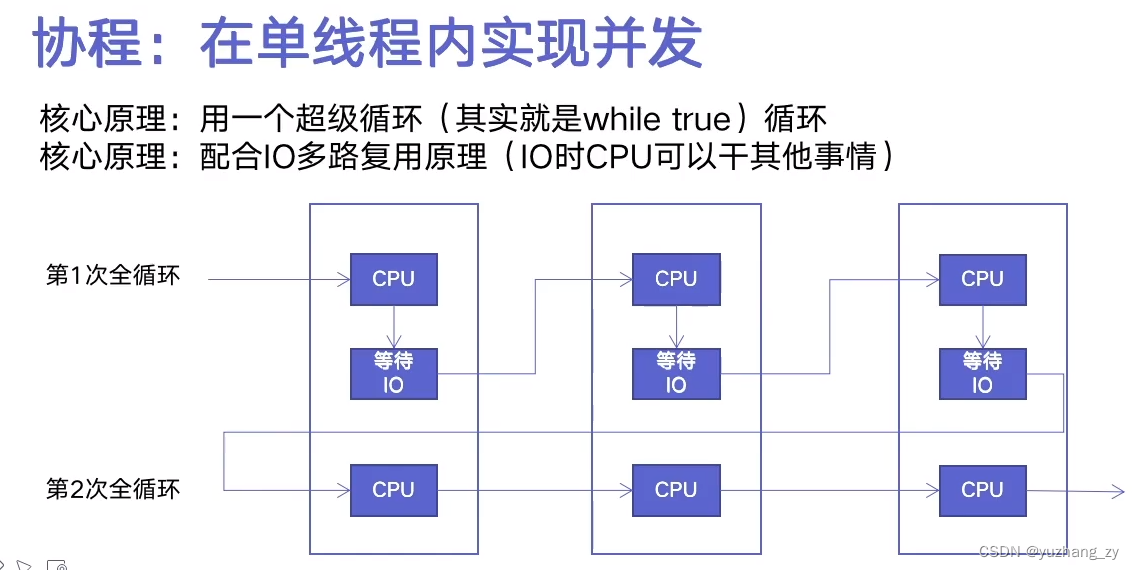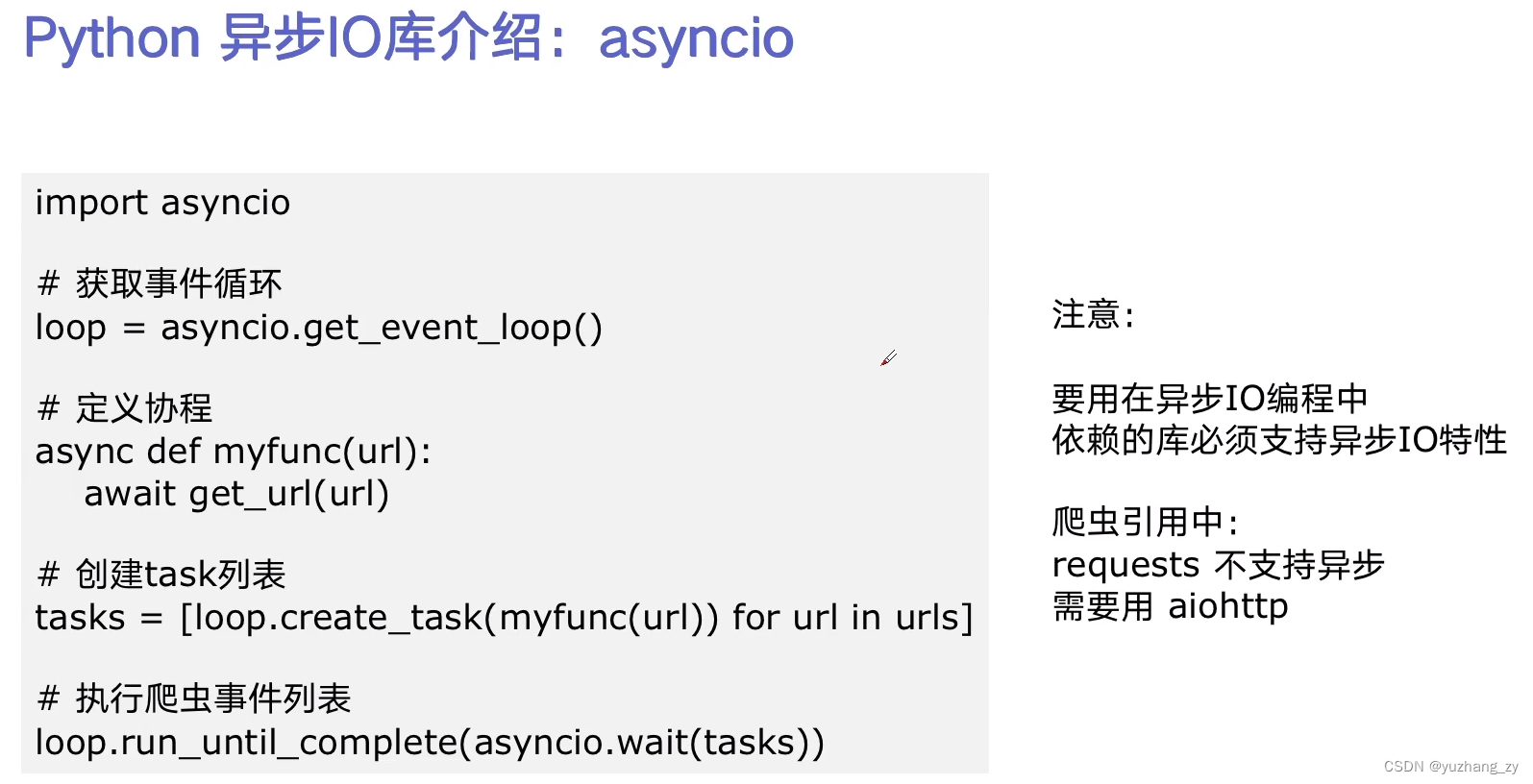``import asyncioimport timeimport aiohttpimport blog_spider  # 协程就是在异步IO中执行的函数, async需要使用超级循环进行调度async def async_craw(url: str):    print("craw url: ", url)    async with aiohttp.ClientSession() as session:        async with session.get(url) as resp:            # await的时候超级循环不会等待而是切换到下一个循环            result = await resp.text()            print(f"craw url:{url}, {len(result)}") loop = asyncio.get_event_loop()tasks = [loop.create_task(async_craw(url)) for url in blog_spider.urls]start = time.time()loop.run_until_complete(asyncio.wait(tasks))end = time.time()print("use time seconds: ", end - start)``

# 十二. 在异步IO中使用信号量控制spider并发度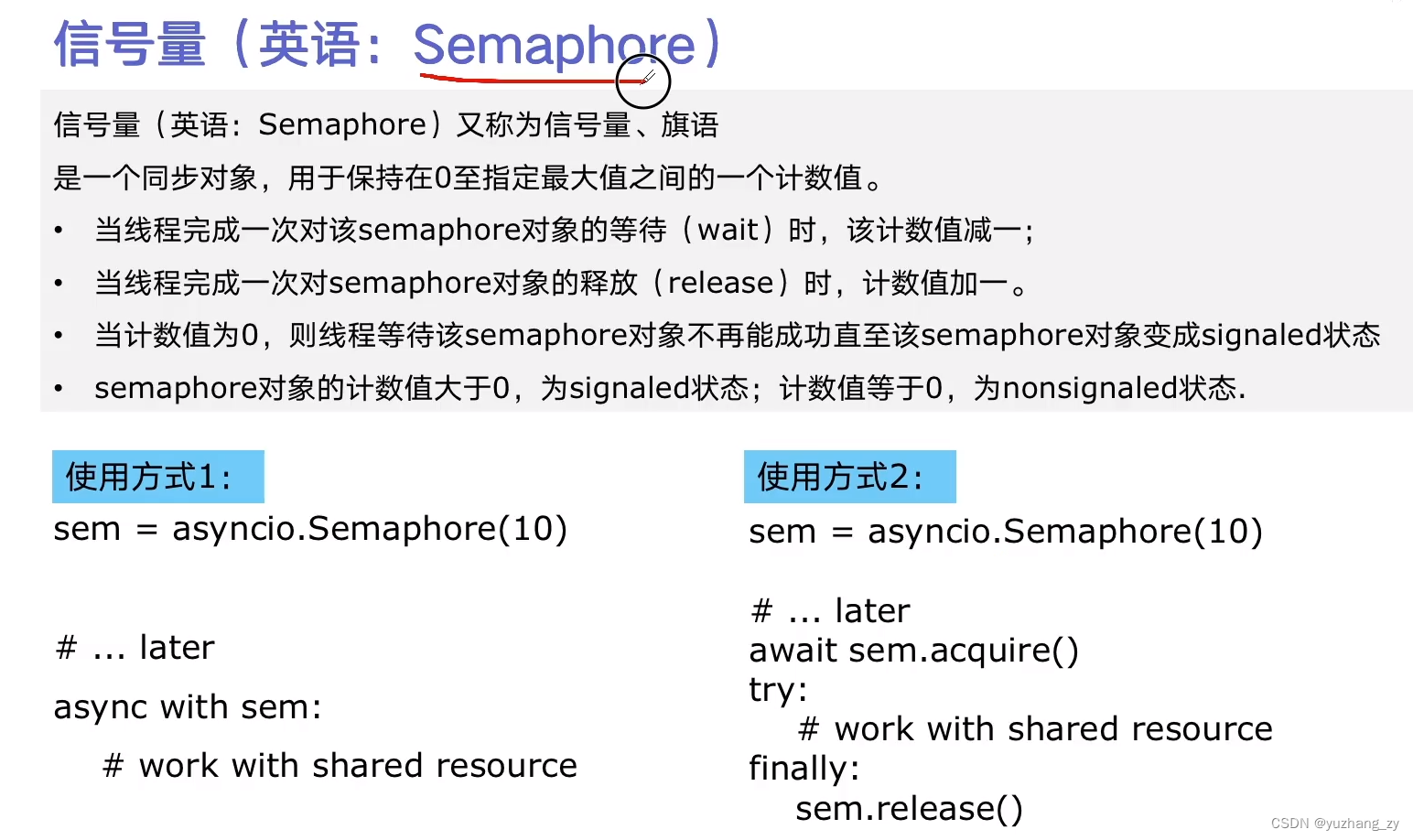``import asyncioimport timeimport aiohttpimport blog_spider # 控制并发度semaphore = asyncio.Semaphore(10)  async def async_craw(url: str):    # 在semaphore 代码内的都是在semaphore 信号量的控制内, 由它来控制并发    async with semaphore:        print("craw url: ", url)        async with aiohttp.ClientSession() as session:            async with session.get(url) as resp:                result = await resp.text()                # 休眠5s                await asyncio.sleep(5)                print(f"craw url:{url}, {len(result)}")  loop = asyncio.get_event_loop()tasks = [loop.create_task(async_craw(url)) for url in blog_spider.urls]start = time.time()loop.run_until_complete(asyncio.wait(tasks))end = time.time()print("use time seconds: ", end - start)``